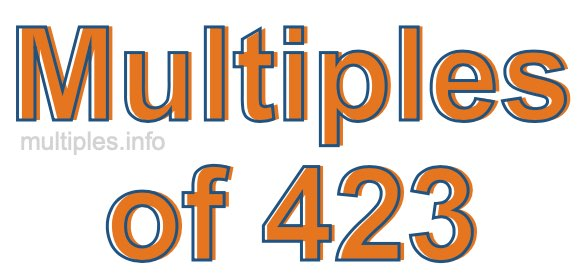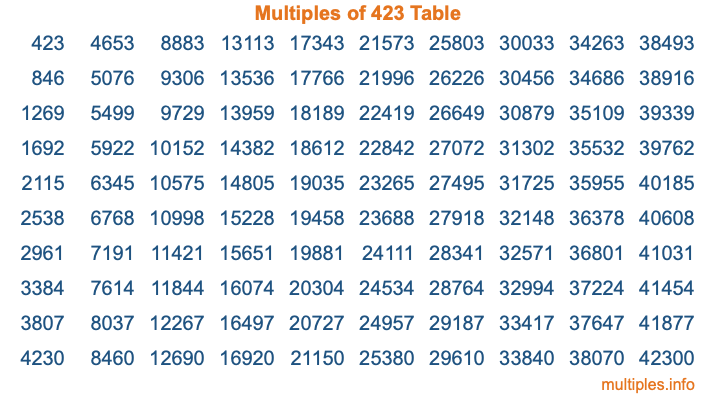Multiples of 423Welcome to the Multiples of 423 page. Here we will first teach you everything you will ever need to know about the multiples of 423, and then give you a study guide summary of everything we taught you to make sure you remember it all. Use this page to look up facts and learn information about the multiples of 423. This page will make you a multiples of four hundred twenty-three expert!

Definition of Multiples of 423
Multiples of 423 are all the numbers that when divided by 423 equal an integer. Each of the multiples of 423 are called a multiple. A multiple of 423 is created by multiplying 423 by an integer.

Therefore, to create a list of multiples of 423, you start with 1 multiplied by 423, then 2 multiplied by 423, then 3 multiplied by 423, and so on for as long as you want. Thus, the list of the first five multiples of 423 is 423, 846, 1269, 1692, and 2115. To see a larger list of multiples of 423, see the printable image of Multiples of 423 further down on this page. We also have a category where you can choose any nth multiple of 423.

Multiples of 423 Checker
The Multiples of 423 Checker below checks to see if any number of your choice is a multiple of 423. In other words, it checks to see if there is any number (integer) that when multiplied by 423 will equal your number. To do that, we divide your number by 423. If the the quotient is an integer, then your number is a multiple of 423.

Is  a multiple of 423?

Least Common Multiple of 423 and ...
A Least Common Multiple (LCM) is the lowest multiple that two or more numbers have in common. This is also called the smallest common multiple or lowest common multiple and is useful to know when you are adding our subtracting fractions. Enter one or more numbers below (423 is already entered) to find the LCM.

Check out our LCM Calculator if you need more details about the Least Common Multiple or if you need the LCM for different numbers for adding and subtraction fractions.

nth Multiple of 423
As we stated above, 423 is the first multiple of 423, 846 is the second multiple of 423, 1269 is the third multiple of 423, and so on. Enter a number below to find the nth multiple of 423.

th multiple of 423

Multiples of 423 vs Factors of 423
423 is a multiple of 423 and a factor of 423, but that is where the similarities end. All postive multiples of 423 are 423 or greater than 423. All positive factors of 423 are 423 or less than 423.

Below is the beginning list of multiples of 423 and the factors of 423 so you can compare:

Multiples of 423: 423, 846, 1269, 1692, 2115, etc.

Factors of 423: 1, 3, 9, 47, 141, 423

As you can see, the multiples of 423 are all the numbers that you can divide by 423 to get a whole number. The factors of 423, on the other hand, are all the whole numbers that you can multiply by another whole number to get 423.

It's also interesting to note that if a number (x) is a factor of 423, then 423 will also be a multiple of that number (x).

Multiples of 423 vs Divisors of 423
The divisors of 423 are all the integers that 423 can be divided by evenly. Below is a list of the divisors of 423.

Divisors of 423: 1, 3, 9, 47, 141, 423

The interesting thing to note here is that if you take any multiple of 423 and divide it by a divisor of 423, you will see that the quotient is an integer.

Multiples of 423 Table
Below is an image of the first 100 multiples of 423 in a table. The table is in chronological order, column by column. The first column has the first ten multiples of 423, the second column has the next ten multiples of 423, and so on.The Multiples of 423 Table is also referred to as the 423 Times Table or Times Table of 423. You are welcome to print out our table for your studies.

Negative Multiples of 423
Although not often discussed or needed in math, it is worth mentioning that you can make a list of negative multiples of 423 by multiplying 423 by -1, then by -2, then by -3, and so on, to get the following list of negative multiples of 423:

-423, -846, -1269, -1692, -2115, etc.

Multiples of 423 Summary
Below is a summary of important Multiples of 423 facts that we have discussed on this page. To retain the knowledge on this page, we recommend that you read through the summary and explain to yourself or a study partner why they hold true.

There are an infinite number of multiples of 423.

A multiple of 423 divided by 423 will equal a whole number.

423 divided by a factor of 423 equals a divisor of 423.

The nth multiple of 423 is n times 423.

The largest factor of 423 is equal to the first positive multiple of 423.

423 is a multiple of every factor of 423.

423 is a multiple of 423.

A multiple of 423 divided by a divisor of 423 equals an integer.

423 divided by a divisor of 423 equals a factor of 423.

Any integer times 423 will equal a multiple of 423.

Multiples of a Number
Here you can get the multiples of another number, all with the same attention to detail as we did for multiples of 423 on this page.

Multiples of
Multiples of 424
Did you find our page about multiples of four hundred twenty-three educational? Do you want more knowledge? Check out the multiples of the next number on our list!